Electron. J. Diff. Equ., Vol. 2010(2010), No. 56, pp. 1-16.

### Existence and multiplicity of solutions to elliptic problems with discontinuities and free boundary conditions Sabri Bensid, Sidi Mohammed Bouguima

Abstract:
We study the nonlinear elliptic problem with discontinuous nonlinearity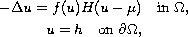where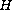is the Heaviside unit function,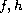are given functions and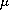is a positive real parameter. The domain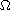is the unit ball in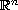with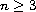. We show the existence of a positive solutionand a hypersurface separating the region where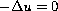from the region where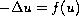. Our method relies on the implicit function theorem and bifurcation analysis.

Submitted February 22, 2010. Published April 19, 2010.
Math Subject Classifications: 34R35, 35J25.
Key Words: Green function; maximum principle; bifurcation; free boundary problem.

Show me the PDF file (273 KB), TEX file, and other files for this article.Sabri Bensid Department of Mathematics, Faculty of Sciences University of Tlemcen, B.P. 119, Tlemcen 13000, Algeria email: edp_sabri@yahoo.fr Sidi Mohammed Bouguima Department of Mathematics, Faculty of Sciences University of Tlemcen, B.P. 119, Tlemcen 13000, Algeria email: bouguima@yahoo.fr